# Shape Puzzles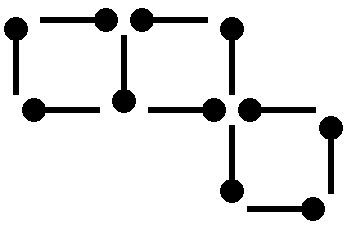A Perfect Match
In this diagram 11 matches make 3 squares. Your challenge is to move 3 matches to show 2... Try Puzzle >>As Easy As Pi
Cut up the Greek letter Pi below into five pieces as shown. Re-arrange the five pieces... Try Puzzle >>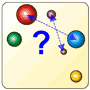You have six beads placed in a plane. The object is to find two pairs of equidistant beads.... Try Puzzle >>Circling The Square
In the diagram below, each circle is just big enough to touch all four sides of the square.... Try Puzzle >>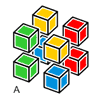Color Cubes
There are nine compositions (A to I) of eight colored cubes. Find two identical compositions.... Try Puzzle >>Crushed Dice
Sam wants to make some dice. But only one of these nets will make dice correctly. Which one?... Try Puzzle >>Cube Nets
Which of these "Nets" will make a Cube? You can print this page, cut them... Try Puzzle >>Cubic Outlines
How many outlines of triangles of all sizes and shapes can you trace in the figure? Try Puzzle >>Cut a 3x3 Cube
Imagine a 3x3x3 cube. How many cuts do we need to break it into 27 1x1x1 cubes? A cut... Try Puzzle >>Cut the Cross
With two straight lines, cut the Swiss Cross into four congruent pieces (same size and shape)... Try Puzzle >>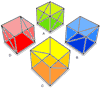Four Cubes Outlines
In the illustration four flat cube-like shapes are shown. Their patterns are drawn with bold... Try Puzzle >>Game For A Half
If you cut the shaded shape A into two pieces in a certain way, you can then make all the other... Try Puzzle >>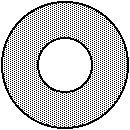Horace and the Doughnut
Hungry Horace has bought a large doughnut to share with his friends at a party. The doughnut... Try Puzzle >>Matches Of The Day
This diagram shows 3 squares and 2 triangles: Rearrange the matches to make 5 squares... Try Puzzle >>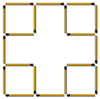Matchstick Frame
There are five squares (one 3x3 and four 1x1) formed with 20 matchsticks, as shown in the illustration.... Try Puzzle >>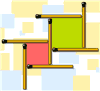One More Square
Seven matchsticks form two squares as shown in the illustration. Move three matchsticks to... Try Puzzle >>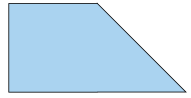One Square and a Half
The figure shown represents a square placed next to another square of the same size but cut... Try Puzzle >>Overlapping Squares
The picture below was made up from four squares stuck one upon another. I am sure you can see... Try Puzzle >>Parted Circle
You have nine circles (divided into 16 smaller sectors). Each has a different pattern of holes.... Try Puzzle >>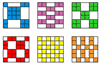Parted Square
You have six squares (divided into 6x6 smaller squares). Each has a different pattern of... Try Puzzle >>Shapely Sequence
This is a sequence of shapes. Try to sketch what shape is next, then see our solution. Try Puzzle >>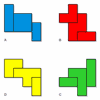Surplus Tetramino
If you re-assemble the pieces of each of the four compositions shown below, three of them will... Try Puzzle >>Ten Balls in Five Lines
Place 10 balls in 5 lines in such a way that each line has exactly 4 balls on it. Try Puzzle >>Time For T
A tea-time jigsaw for you to try this week. Sorry I forgot the biscuits! Try Puzzle >>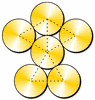Triangle Man Puzzle
Six coins make the Triangle Man shown in the illustration. The coins form three identical... Try Puzzle >>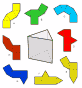Unfold the Prism
Eight folding patterns are scattered around a triangular prism as shown in the illustration.... Try Puzzle >>